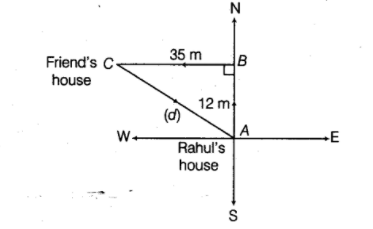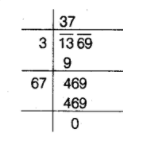# Rahul walks 12m North from his house and turns

Question:

Rahul walks 12m North from his house and turns West to walk 35m to reach his friend’s house. While returning, he walks diagonally from his

friend’s house to reach back to his house. What distance did he walk, while returning?

Solution:

Let Rahul walked $x \mathrm{~m}$, while returning home.In $\Delta A B C$, by using Pythagoras theorem, we get$A C^{2}=A B^{2}+B C^{2}$

$\Rightarrow \quad A C^{2}=(12)^{2}+(35)^{2}$

$\Rightarrow \quad A C^{2}=144+1225=1369$

$\Rightarrow \quad A C=\sqrt{1369}$

$\Rightarrow \quad A C=37 \mathrm{~m}$

Hence, Rahul walked $37 \mathrm{~m}$ distance for returning to his house diagonally.